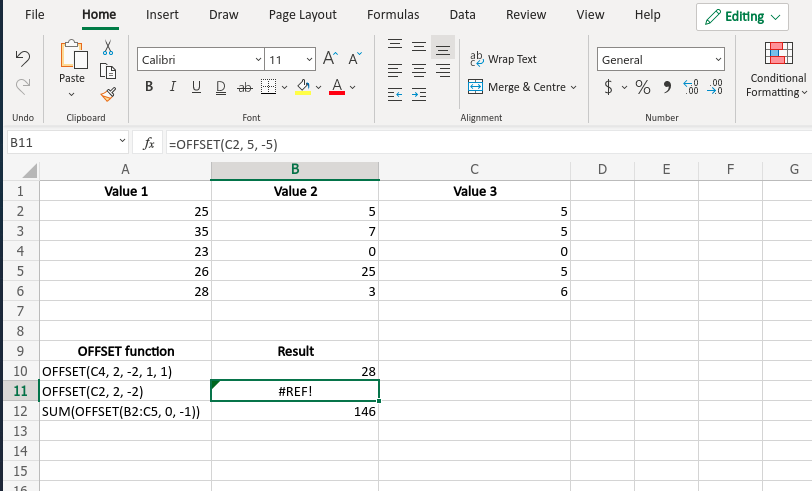# OFFSET Function in Excel With Examples

• Last Updated : 27 May, 2021

Excel contains many useful formulas and functions that make it more and more useful and at the same time user-friendly. Such a function is the OFFSET() function. In many cases, this function is also used inside another function. This function basically returns a reference of a single cell or a range of cells depending on the input. With the help of this function, we can traverse from one cell to another cell. Moreover, the user can specify the number of rows and columns to be returned.

This function is a volatile function that also works according to its name. It returns a reference of the desired element(s) that is(are) ‘offset’ of some distance from the given starting point. This can be used within some functions to make tasks easier.

Syntax:

```OFFSET(reference, rows, cols, [height], [width])

-->Here the [height] and [width] are optional.```

This function takes a valid reference, rows, and columns(To know how much distance the function will traverse), and height and width(that describes the height and width of output reference) as its arguments and returns the desired reference of the cell or the range of cells. These arguments are discussed below elaborately.

Arguments:

• reference (Required): This is the reference of the starting point or the base of the OFFSET() function and this reference must be provided by the user. The reference must be valid i.e. a reference of a single cell or a reference to a range of adjacent cells.
• rows (Required): This argument contains the number of rows(up or down) that are used by the OFFSET() function to traverse up or down. Again this argument must be provided by the user. This value may be negative or positive. A negative value denotes that the function has to traverse upwards and a positive value denotes traversing downwards.
• cols (Required): This argument contains the number of columns provided by the user. With the help of this argument OFFSET(), function traverse left or right of the starting point. This value may also be negative or positive. A negative value means traversing to the left and a positive value means traversing to the right.
• [height] (Optional): This is the height of the reference to be returned by the OFFSET() function. Basically, this value denotes the number of rows of the returned reference. This value must be a positive number. But this is an optional argument. If this argument is omitted, OFFSET() returns a reference of the same height as the ‘reference’(starting point).
• [width] (Optional): This is the width of the reference to be returned by the OFFSET() function. Basically, this value denotes the number of columns of the returned reference. This value must also be a positive number. But again this is an optional argument. If this argument is omitted, OFFSET() returns a reference of the same width as the ‘reference’(starting point).
`Note: The number of rows and columns must not exceed the height and width of the worksheet.`

Return Value: This function naturally returns a reference to a range of cells(sometimes a single cell depending on the input arguments) of a certain height and width either same as the ‘reference’ argument or as the user has provided.

Example:

An Excel sheet has been taken as an example and the OFFSET function has been used in several formats.

OFFSET function has been applied to the above table.

Output:My Personal Notes arrow_drop_up